### Confidence Limits for a Hazard Ratio

Let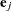be the jth unit vector—that is, the jth entry of the vector is 1 and all other entries are 0. The hazard ratio for the explanatory variable with regression coefficient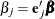is defined as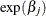. In general, a log-hazard ratio can be written as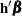, a linear combination of the regression coefficients, and the hazard ratio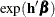is obtained by replacingwith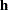.

#### Point Estimate

The hazard ratio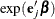is estimated by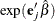, where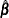is the maximum likelihood estimate of the.

#### Wald’s Confidence Limits

The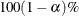confidence limits for the hazard ratio are calculated as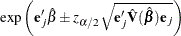where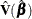is estimated covariance matrix, and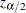is the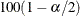percentile point of the standard normal distribution.

#### Profile-Likelihood Confidence Limits

The construction of the profile-likelihood-based confidence interval is derived from the asymptoticdistribution of the generalized likelihood ratio test of Venzon and Moolgavkar (1988). Suppose that the parameter vector is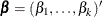and you want to compute a confidence interval for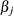. The profile-likelihood function for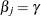is defined as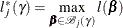where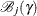is the set of allwith the jth element fixed at, and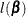is the log-likelihood function for. If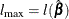is the log likelihood evaluated at the maximum likelihood estimate, then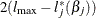has a limiting chi-square distribution with one degree of freedom ifis the true parameter value. Let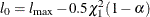, where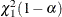is thepercentile of the chi-square distribution with one degree of freedom. A% confidence interval foris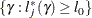The endpoints of the confidence interval are found by solving numerically for values ofthat satisfy equality in the preceding relation. To obtain an iterative algorithm for computing the confidence limits, the log-likelihood function in a neighborhood ofis approximated by the quadratic function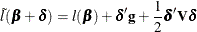where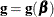is the gradient vector and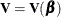is the Hessian matrix. The increment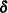for the next iteration is obtained by solving the likelihood equations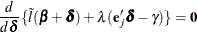whereis the Lagrange multiplier,is the jth unit vector, andis an unknown constant. The solution is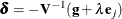By substituting thisinto the equation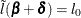, you can estimateas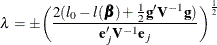The upper confidence limit foris computed by starting at the maximum likelihood estimate ofand iterating with positive values ofuntil convergence is attained. The process is repeated for the lower confidence limit, using negative values of.

Convergence is controlled by valuespecified with the PLCONV= option in the MODEL statement (the default value ofis 1E–4). Convergence is declared on the current iteration if the following two conditions are satisfied: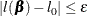and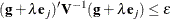The profile-likelihood confidence limits for the hazard ratioare obtained by exponentiating these confidence limits.